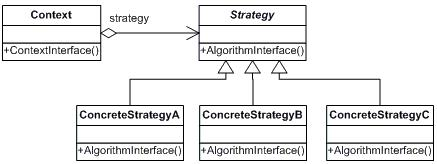# Design Patterns : Strategy pattern

## Motivation

There are situations where the classes differ only by their behaviour, and on such situations it is advisable to isolate the algorithms into separate classes in order to have the ability to select different algorithms at the runtime depending on the user needs/client inputs.

## Intent

• “Define a family of algorithms, encapsulate each one, and make them interchangeable. Strategy lets the algorithm vary independently from the clients that use it.” [Gamma, p315]
• Capture the abstraction in an interface, bury implementation details in derived classes.

## Diagram## Code Sample

`//StrategyExample test application`
`class StrategyExample {`
`    public static void main(String[] args) {`
`         Context context;`
`        // Three contexts following different strategies`
`        context = new Context(new FirstStrategy());`
`        context.execute();`
`        context = new Context(new SecondStrategy());`
`        context.execute();`
`        context = new Context(new ThirdStrategy());`
`        context.execute();`
`    }`
`}`
`// The classes that implement a concrete strategy should implement this`
`// The context class uses this to call the concrete strategy`
`interface Strategy {`
`    void execute();`
`}`
`// Implements the algorithm using the strategy interface`
`class FirstStrategy implements Strategy {`
`    public void execute() {`
`        System.out.println("Called FirstStrategy.execute()");`
`    }`
`}`
`class SecondStrategy implements Strategy {`
`    public void execute() {`
`        System.out.println("Called SecondStrategy.execute()");`
`    }`
`}`
`class ThirdStrategy implements Strategy {`
`    public void execute() {`
`        System.out.println("Called ThirdStrategy.execute()");`
`    }`
`}`
`// Configured with a ConcreteStrategy object and maintains a reference to a Strategy object`
`class Context {`
`    Strategy strategy;`
`    // Constructor`
`    public Context(Strategy strategy) {`
`        this.strategy = strategy;`
`    }`
`    public void execute() {`
`        this.strategy.execute();`
`    }`
`}`